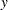Output Data Sets

The KRIGE2D procedure produces two data sets: the OUTEST=SAS-data-set and the OUTNBHD=SAS-data-set. These data sets are described as follows.

OUTEST=SAS-data-set

The OUTEST= data set contains the kriging predictions and the associated standard errors. The OUTEST= data set contains the following variables:

• ESTIMATE, which is the kriging prediction for the current variable.

• GXC, which is thecoordinate of the grid point at which the kriging prediction is made.

• GYC, which is thecoordinate of the grid point at which the kriging prediction is made.

• LABEL, which is the label for the current PREDICT/MODEL combination that produces the kriging prediction. If you do not specify a label, default labels of the form Predj.Modelk are used.

• NPOINTS, which is the number of points used in the prediction. This number varies for each grid point if local kriging is performed.

• STDERR, which is the standard error of the kriging predict.

• VARNAME, which is the variable name.

OUTNBHD=SAS-data-set

When you specify the RADIUS= option or the NUMPOINTS= option in the PREDICT statement, local kriging is performed. Local kriging is simply ordinary kriging at a given grid location, using only those data points in a neighborhood defined by the RADIUS= value or the NUMPOINTS= value.

The OUTNBHD= data set contains one observation for each data point in each neighborhood. Hence, this data set can be large. For example, if the grid specification results in 1,000 grid points and each grid point has a neighborhood of 100 points, the resulting OUTNBHD= data set contains 100,000 points.

The OUTNBHD= data set contains the following variables:

• GXC, which is thecoordinate of the grid point.

• GYC, which is thecoordinate of the grid point.

• ID, which is the ID variable value or observation. number of the current data point

• LABEL, which is the label for the current PREDICT/MODEL combination. If you do not specify a label, default labels of the form Predj.Modelk are used.

• NPOINTS, which is the number of points used in the prediction.

• XC, which is thecoordinate of the current data point.
• YC, which is thecoordinate of the current data point.